# Arduino-ESP WiFi Integration

Use the program to manage your ESP module with simple commands. This will allow you to access the Internet for any Arduino product.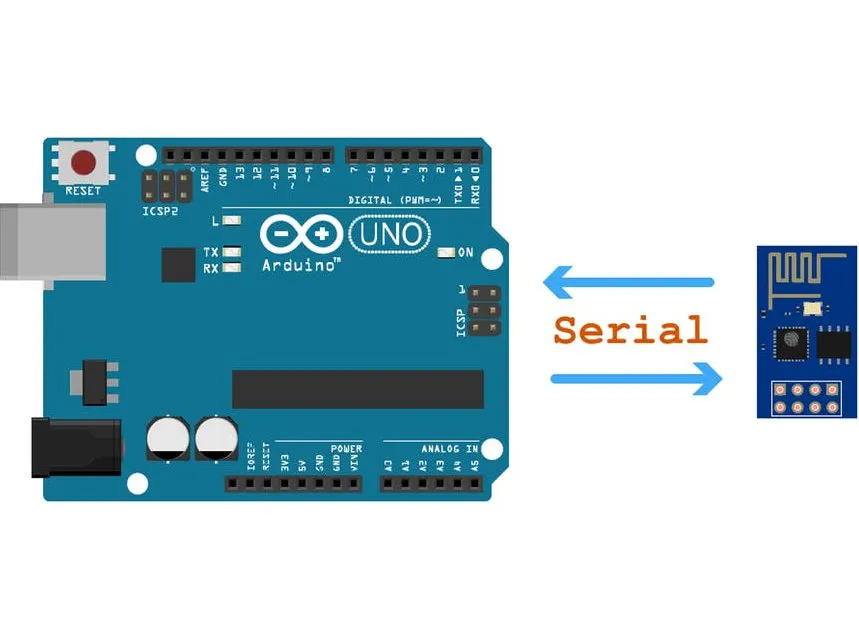## Things used in this project

### Hardware componentsArduino UNO & Genuino UNO
×1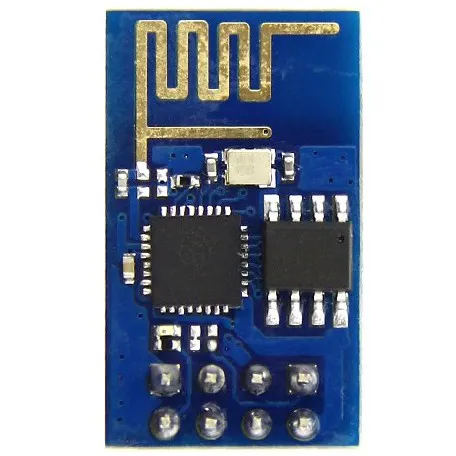Espressif ESP8266 ESP-01
×1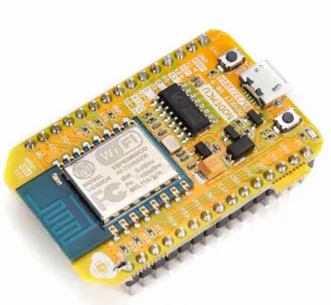NodeMCU ESP8266 Breakout Board
×1

### Software apps and online servicesArduino IDE

## Schematics

### Circuit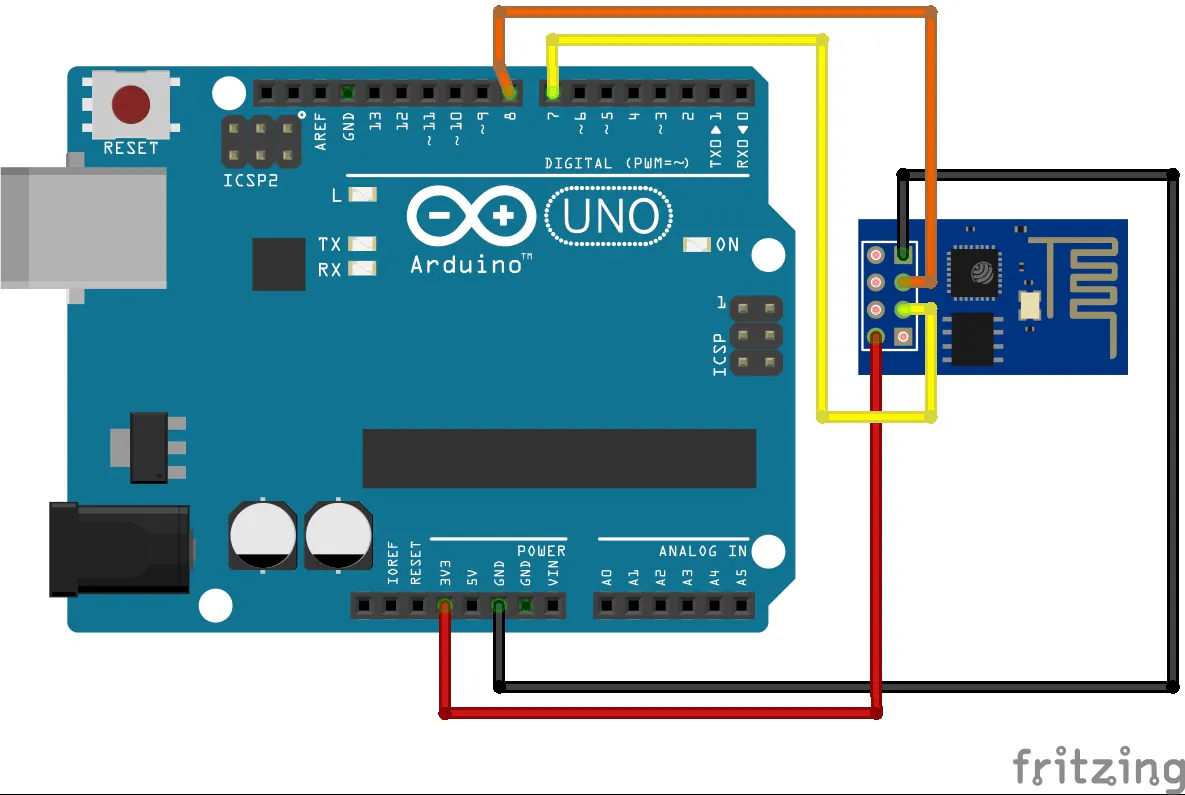### nodeMCU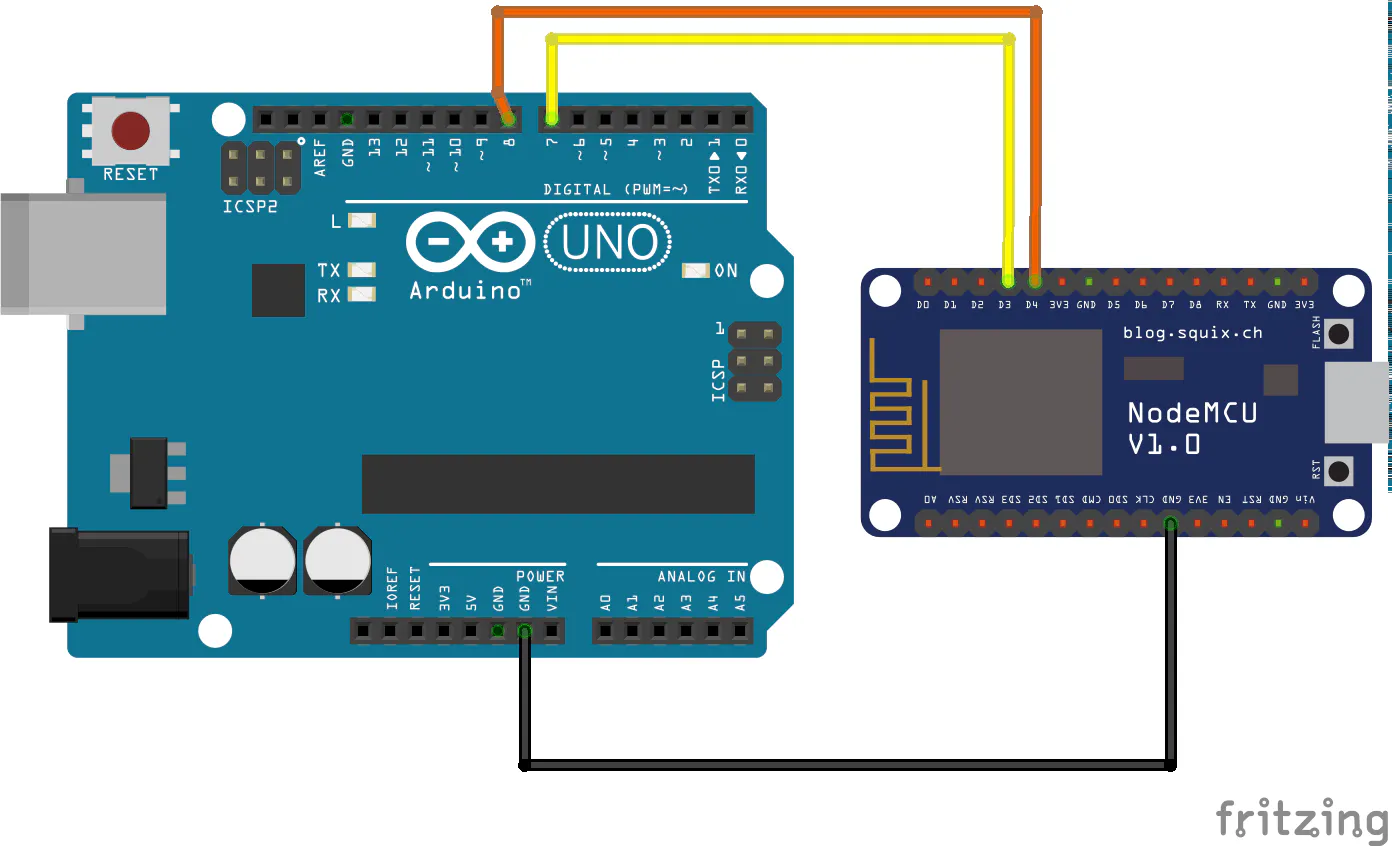## Code

### arduino

Arduino
```#include<SoftwareSerial.h>

SoftwareSerial esp(7, 8);

String wrong;   //Wrong respons buffer

void setup()
{
esp.begin(9600);
Serial.begin(9600);   //start the serial port

String arg[]={"WifiSSID","WIFIPASSWD"}; //create a String array for arguments
wifi(1,arg);    //connect to a network
}

void loop()
{
if (esp.available())
}

{
String buff = esp.readStringUntil(char(244));   //read datas to the "buff" until the character ¶ character (An arbitrary character)
buff.replace(String(char(244)),""); //delet this char from buffer
byte id=byte(buff.charAt(0)); //get the id from the first char of buffer
buff.remove(0,1); //remove the first char. we don't need any more
if ((byte(task.charAt(0)) + id) == 255)  //if the task id and response id are inverts
{
return buff;    //return
}
else
{
Serial.println("Old datas:\n");   //else this are old datas. Read to wrong
wrong=buff.substring(1);
Serial.println(wrong);
}
return "-1";  //this can not happen
}

void wifi(byte id,String data[])  //function wifi with arguments
{
String output=String(char(id)); //add the converted id to output
for(int k=0;k<sizeof(data);k++)   //devide arguments with a "\t" char
output+=data[k]+"\t";
esp.println(output);  //send datas to esp
}
void wifi(byte id)    //This is the same as the previous one, without arguments
{
String output=String(char(id));
esp.println(output);
}
```

### esp

Arduino
```#include <SoftwareSerial.h>
#include <ESP8266WiFi.h>
#include <ESP8266HTTPClient.h>
#include <WiFiClient.h>

SoftwareSerial ard(0,2);

void setup()
{
WiFi.mode(WIFI_STA); //start wifi as station
Serial.begin(9600);
ard.begin(9600);    //start serial port
}

void loop()
{
if (ard.available())
}

{
task = buff.charAt(0); //set up the id
int ind = 1; //indexing value
for (int l = 0; l < buff.length(); l++)
{
if (buff.charAt(l) == '\t')
ind++;
else
}
byte id = byte(task.charAt(0));    //set up id
byte invert = ~id;    //create invert data
Serial.print("id: "); Serial.print(id); Serial.print("\t"); //print incomeing datas
Serial.print("Invert: "); Serial.println(invert);   //not necessary, just for debuging
String resp = String(char(invert)); //add invert id to response
switch (id)   //check for command
{
case 1:
{
//WiFi client
Serial.print("Resp:\t"); Serial.println(resp.substring(1));
break;
}
case 2:
{
//WiFi AP
break;
}
case 3:
{
//scan network
resp += scan();
Serial.print("Resp:\t"); Serial.println(resp.substring(1));
break;
}
case 4:
{
//is connected?
if (WiFi.status() == WL_CONNECTED)
resp += "connected";
else
resp += "disconnected";
Serial.print("Resp:\t"); Serial.println(resp.substring(1));
break;
}
case 9:
{
WiFi.disconnect();
resp+="ok";
break;
}
case 21:
{
//http client
Serial.print("Resp:\t"); Serial.println(resp.substring(1));
break;
}
}
ard.println(resp);    //send datas
}

{
Serial.println("Wifi connect to");
WiFi.mode(WIFI_STA);  //set up wifi as station
for (int tim = 0; tim < 30; tim++)
{
if (WiFi.status() == WL_CONNECTED)
break;
else
delay(500);
}
//try for 15 seconds connect to network
Serial.println(WiFi.localIP());
if (WiFi.status() == WL_CONNECTED)
return (String(WiFi.localIP()) + "." + String(WiFi.localIP()) + "." + String(WiFi.localIP()) + "." + String(WiFi.localIP()));
else
return "0";
//if we succeed, we will return with the ip address, if we do not return with 0
}

String scan()
{

int n = WiFi.scanNetworks();
if (n == 0)
return "0 networks availabele";
else
{
String ret = "";
for (int i = 0; i < n; ++i) {
ret += WiFi.SSID(i);
ret += "\t";
ret += "\t";
ret += ((WiFi.encryptionType(i) == ENC_TYPE_NONE) ? "Opened" : "Closed");
ret += "\n";
}
return ret;
}
}

String http_client(String URL)
{
if ((WiFi.status() == WL_CONNECTED))
{
WiFiClient client;
HTTPClient http;
if (http.begin(client, URL))
{
int httpCode = http.GET();
if (httpCode > 0)
{
if (httpCode == HTTP_CODE_OK || httpCode == HTTP_CODE_MOVED_PERMANENTLY)
{
http.end();
return payload;   //return with the source code
}
}
else
return "False request";
}
}
else
{
return "Not connected";
}
}
```

## Credits

### Turai Botond

4 projects • 16 followers